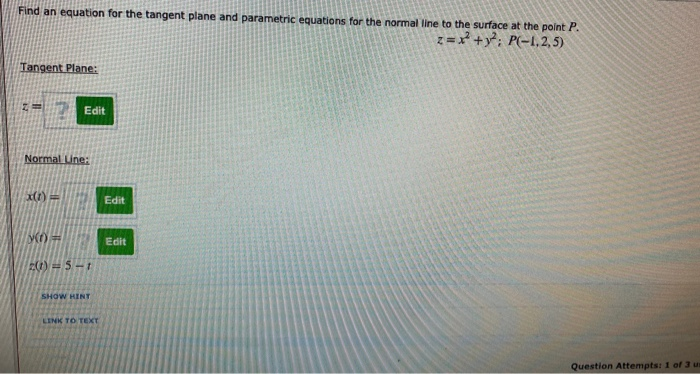# Question Solved1 AnswerFind an equation for the tangent plane and parametric equations for the normal line to the surface at Point P: Find an equation for the tangent plane and parametric equations for the normal line to the surface at the point P. z=x +y?: P(-1.2,5) Tangent Plane: Edit Normal Une: (1) = Edit VO Edit (i) = 5-1 SHOW HINT LINK TO TEXT Question Attempts: 1 of 3Find an equation for the tangent plane and parametric equations for the normal line to the surface at Point P:Transcribed Image Text: Find an equation for the tangent plane and parametric equations for the normal line to the surface at the point P. z=x +y?: P(-1.2,5) Tangent Plane: Edit Normal Une: (1) = Edit VO Edit (i) = 5-1 SHOW HINT LINK TO TEXT Question Attempts: 1 of 3
More
Transcribed Image Text: Find an equation for the tangent plane and parametric equations for the normal line to the surface at the point P. z=x +y?: P(-1.2,5) Tangent Plane: Edit Normal Une: (1) = Edit VO Edit (i) = 5-1 SHOW HINT LINK TO TEXT Question Attempts: 1 of 3